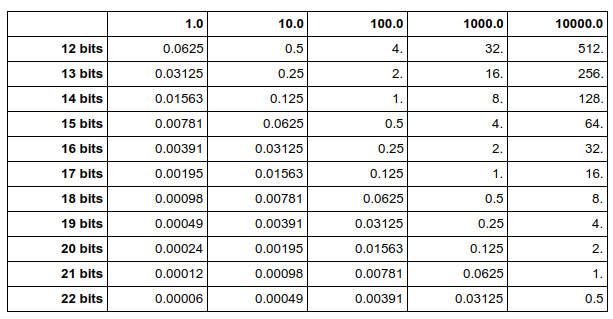# Making floating point numbers smaller

When the goal is to make a 64kB executable (or less!), many unexpected issues arise. Floating point numbers are found everywhere: position of objects in the world, position of the camera, constants for the effets, colors in the texture generator, etc. In practice, we often don’t need as much precision as offered by floats. Can we take advantage of that to pack more data in a smaller space?

Whether an object is 2.2 or 2.21 meters high, is not meaningful. The goal here is to reduce the amount of space used by those numbers. A float takes 4 bytes (8 bytes for a double). This can be reduced a bit with compression, but when there are thousands of them, it’s still quite big. We can do better.

## A naïve solution

Suppose we have some numbers between 0 and 1000, and we need a 0.1 precision. We could store those numbers as integers between 0 and 10000 and then divide by 10. Whether we use 32 bit or 16 bit integers in the code doesn’t make a difference: since we don’t use their full range of values, all these integers start with leading 0s. The compression code will detect such repetitive 0s and use around 13 bits per number in both cases.

The problem with this solution is that we need some processing in the runtime code. Each time we use a number, we have to convert it to a float and divide it by 10. If all our data is in a same place, we can loop over it. But if we have numbers all over our code base, we’ll also need processing code in all those places. This simple operation can be cumbersome and expensive in terms of space.

It turns out we can get rid of the processing, and use directly floating point numbers.

## A note on IEEE floats

Floats are stored using the IEEE 754 standard. Some of them have a binary representation that contains lots of 0 and compress better than others.

Let’s look at two examples using a binary representation. The IEEE representation is not exactly the same as in the example below (it has to store the exponent), but almost.

• 6.25 -> 110.01
• 6.3 -> 110.010011…

In fact, 6.3 has no exact representation in base 2: the number stored is an approximation, and it would require an infinite number of digits to represent 6.3. On the other hand, the binary representation for 6.25 is compact and exact.

If we’re optimizing for size, we should prefer numbers like 6.25, that have a compact binary representation. For example, 0.125, 0.5, 0.75, 0.875 have at most 3 digits in binary after the decimal mark. The binary representation will have a lot of 0s at the end of the number, which will compress really well. The great thing is that we don’t need processing code anymore because we’re still using standard floats.

To better understand IEEE representation, try some tools to visualize the floats. You’ll see how removing the last 1s will reduce the precision.

## How much precision do we need?

Floats are much more precise for values around 0. As our numbers get bigger, we’ll have less and less precision (or we’ll need more bits).

The table below is useful to check how much precision is needed. It tells you the worst error to expect based on the number of bits, and the scale of the input numbers. For example, if the input numbers are around 100 and we use 16 bits per float, the error will be at most 0.25. If we want the error to be less than 0.01, we need 21 bits per float.Of course, each time you add a bit, you divide by two the expected error.

## How to automate it?

An ad hoc solution is to remember this list of numbers and use them in the code when possible: 0.125, 0.25, 0.375, 0.5, 0.625, 0.75, 0.875. An alternative is to use a list of macros from Iñigo Quilez. As Iñigo points out, this is not very elegant. Fortunately, this is hardly a problem because chances are this is not where most of your data lies.

64kB can actually contain a lot of data. Developers often rely on tools and custom editors to quickly modify and iterate on the data. In that case, we can easily use code to truncate the floating point numbers as part of the process.

Here is the function we use to round the binary representation of the floats:

1.

Just pass the float, choose how many bits you want to keep, and you’ll get a new float that will compress much better. If you generate C++ code with that number, be careful when printing it (make sure you print it with enough decimals):

1.

The great thing about this function is that we decide exactly how much precision we want to keep. If we desperately need space at some point, we can try to reduce that number and see what happens.

By applying this technique, we’ve managed to save several kilobytes on our 64kB executable.
Hopefully you will, too.